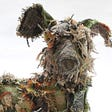# What is deep learning?

Deep learning is a subset of machine learning that involves artificial neural networks.¹ Artificial neural networks were somewhat inspired by the natural neurological systems in animals.² Like an animal nervous system an artificial neural network is made up of many nodes, often called “neurons”. Below you can see an illustration of a biological neuron on top and a simple diagram of a artificial neuron on the bottom, Notice that they have a somewhat similar shape.³ Unlike in an animal, neurons in an artificial neural network communicate with numbers and not with chemical neurotransmitters. Nonetheless deep learning models are capable of doing some things that before, only human brains could do.²

³

# Uses of Deep Learning

There are many things only deep learning models and humans are good at, and sometimes the former does a better job. Artificial neural networks can be highly effect at complex tasks.⁴ They can be used to predict whether someone has a disease by looking at there X-ray photos, they can be used for facial and voice recognition, they are responsible for self driving cars, and they can even play video games at the professional level!² In the image below you can see an example of computer vision.⁵ Furthermore deep learning can be used to replace and outperform traditional machine learning algorithms when the amount of data is large and/or when feature selection is not feasible.⁴ The disadvantages of artificial neural networks is that they require large amounts of data and computational power to train. Now that we have an idea of what they are good for let’s dive into how they work!

# Basic Parts of a Neural Network

There are specialized types of neural networks such as convolutional and recurrent neural networks to name a few, but those are out of the scope of this blog. Here I will go over just the basics of neural networks. I will use a simple network with four layers illustrated below.⁶ The first column of nodes on the left is the input layer, Then there are two hidden layers, and finally an output layer. The input layer holds the inputs and has the same amount of nodes as there are features in the data. The hidden layers create more abstract features from the input data, breaking the data down into perhaps simple edges and shapes from an image or different syllables and phonemes from a sound clip.⁷ The number of nodes in this layer varies depending on the problem. Lastly the output layer gives the output of the model. There will be one neuron here if it a regression task and if it is a classification task there will be neurons numbering the amount of classes in the problem. Those arrows between the layers are outputs and they each have a designated weight. Other than in the input layer, each node has whats called an activation function and a bias. All nodes will have the same activation function as their neighbors in the layer. Now that we know the parts of a network let’s move on to how they are trained!

# Setting Up a Model

The first thing that is done after determining the amount of layers and size of each layer is setting the activation functions. For this example one might use relu functions in the two hidden layers and a sigmoid function in the output layer. The relu function simply returns the input if the input is positive, otherwise it returns zero. The sigmoid function fits a number between -1 and 1. Next the weights between and biases within each neuron are set to a random value. In practice all these things are done as the layers are set up. In this model I am going to set the batch size to one. This means that the model will update itself after each row of data is processed. Finally a loss function is required. This depends on what type of model you are using. For regression it is typically the normal root-mean-squared-error or MRSE. Now that the theoretical model is fully set up it is time to move on to training!

# Training the Model

After a model is built it is time to train it on some data. Essentially training the model is just tuning the weights and biases to make the model effective at its task. Next a matrix of the X values are taken. So we have three input neurons so lets say we have training data with three features and one thousand records, and . In the each iteration of training this will produce a 100x3 matrix. Now begins what is called forward propagation. The dot product of the 100x3 matrix of X values will be taken with respect to a 3x4 matrix of weights I will call , that is four weights generating from each of the three input neurons (since there are four neurons in the first hidden layer). This first dot product I will call , it has a shape of 100x4. Nowis passed through the relu function having the function transform every cell in the matrix turning it into a new matrix I will call . Now acts as a new X and the process repeats until you get the final a value. Then we move onto backward propagation. Backward propagation requires a lot of computation, but is relatively straight forward. What happens is each weight and bias is updated by the partial derivative of the loss function with respect to that weight or bias. There is a variable called the “learning rate” which controls the intensity of how it is updated.

# Sources

1. Wikipedia article on deep learning. https://en.wikipedia.org/wiki/Deep_learning
2. Wikipedia article on artificial neural networks. https://en.wikipedia.org/wiki/Artificial_neural_network
3. Images of neurons. https://www.quora.com/What-is-the-differences-between-artificial-neural-network-computer-science-and-biological-neural-network
4. Blog post on advantages of deep learning posted by Sanbit Mahapatra. https://towardsdatascience.com/why-deep-learning-is-needed-over-traditional-machine-learning-1b6a99177063
5. Image showing computer vision. http://www.image-net.org/challenges/LSVRC/2014/
6. Image of a multilayer perceptron. Imad Dabbura. https://towardsdatascience.com/coding-neural-network-forward-propagation-and-backpropagtion-ccf8cf369f76
7. Youtube 3Blue1Brown. But what is a Neural Network? | Deep learning, chapter 1. https://www.youtube.com/watch?v=aircAruvnKk

--

--

--

Data scientist learning at Flat Iron School

Love podcasts or audiobooks? Learn on the go with our new app.

## TensorFlow Lite is Going to Space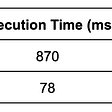## Machine Learning: Which Way to Go?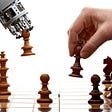## Practical tips for better quantization results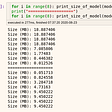## Natural Language Processing Text Classification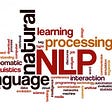## Logistic Regression with Python Using Optimization Function## Are Data Scientists More Like Cats … or Dogs?## More than the mean: Weighting sentence embeddings## Quick Introduction to Bayes Classifier (Naïve)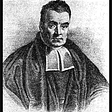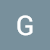## Datascience George

Data scientist learning at Flat Iron School

## Travelusave.com## Modernity of the Seven Deadly Sins